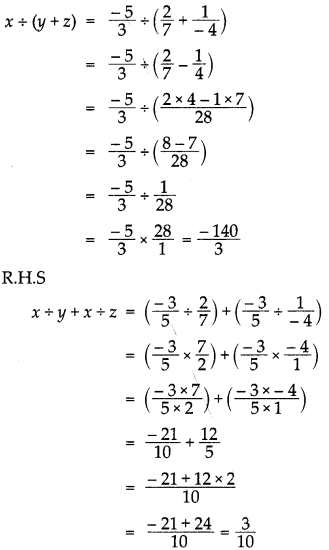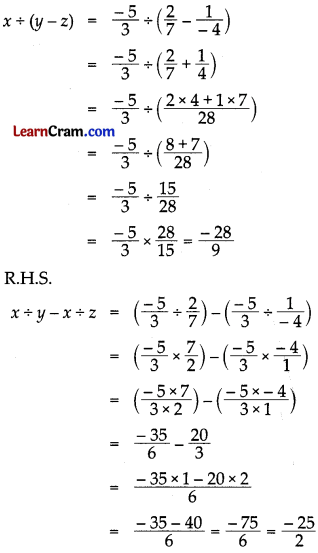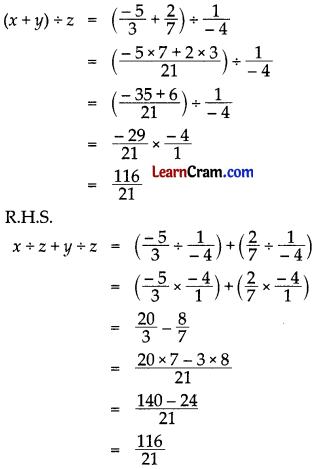# DAV Class 7 Maths Chapter 2 Worksheet 5 Solutions

The DAV Class 7 Maths Book Solutions Pdf and DAV Class 7 Maths Chapter 2 Worksheet 5 Solutions of Operations on Rational Numbers offer comprehensive answers to textbook questions.

## DAV Class 7 Maths Ch 2 WS 5 Solutions

Question 1.
Divide:
(i) $$\frac{2}{5}$$ by $$\frac{-1}{3}$$
$$\frac{2}{5} \div \frac{-1}{3}$$
= $$\frac{2}{5} \times \frac{-3}{1}=\frac{2 \times-3}{5 \times 1}$$
= $$\frac{-6}{5}$$

(ii) $$\frac{-7}{4}$$ by $$\frac{1}{8}$$
$$\frac{-7}{4} \div \frac{1}{8}$$
= $$\frac{-7}{4} \times \frac{8}{1}=\frac{-7 \times 8}{4 \times 1}$$
= $$\frac{-56}{4}$$

(iii) -10 by $$\frac{1}{5}$$
-1 ÷ $$\frac{1}{5}$$
= -1 × 5
= -5

(iv) $$\frac{1}{13}$$ by -2
$$\frac{1}{13}$$ ÷ -2
= $$\frac{1}{13} \times \frac{-1}{2}=\frac{1 \times-1}{13 \times 2}$$
= $$\frac{-1}{26}$$Question 2.
By taking x = $$\frac{3}{4}$$ and y = $$\frac{-5}{6}$$ verify that x ÷ y ≠ y ÷ x
L.H.S = x ÷ y = $$\frac{3}{4} \div \frac{-5}{6}$$
= $$\frac{3}{4} \times \frac{6}{-5}$$
= $$\frac{3 \times 6}{4 \times-5}$$
= $$\frac{18}{-20}=\frac{-18}{20}$$

R.H.S = y ÷ x = $$\frac{-5}{6} \div \frac{3}{4}$$
= $$\frac{-5}{6} \times \frac{4}{3}$$
= $$\frac{-5 \times 4}{6 \times 3}=\frac{-20}{18}$$

They are not same but reciprocal to each other. Hence x ÷ y ≠ y ÷ x

Question 3.
The product of two rational numbers is $$\frac{-3}{7}$$. If one of the number is $$\frac{5}{21}$$, find the other.
Let the other number be x and the given number is $$\frac{5}{21}$$
∴ $$\frac{5}{21}$$ × x = $$\frac{-3}{7}$$
x = $$\frac{-3}{7} \div \frac{5}{21}$$
= $$\frac{-3}{7} \times \frac{21}{5}$$
= $$\frac{-63}{35}=\frac{-9}{5}$$

Hence the other number = $$\frac{-9}{5}$$

Question 4.
With what number should we multiply $$\frac{-36}{35}$$, so that the product be $$\frac{-6}{5}$$ ?
Solution:
Let the required number be x
∴ x × $$\frac{-36}{25}=\frac{-6}{5}$$
⇒ x = $$\frac{-6}{5} \div \frac{-36}{25}$$
= $$\frac{-6}{5} \times \frac{25}{-36}=\frac{5}{6}$$
Hence the required number = $$\frac{5}{6}$$.

Question 5.
By taking x = $$\frac{-5}{3}$$, y = $$\frac{2}{7}$$ and z = $$\frac{1}{-4}$$ verify that:
(i) x ÷ (y + z) ≠ x ÷ y + x ÷ z
L.H.S(ii) x ÷ (y – z) ≠ x ÷ y – x ÷ z
L.H.SL.H.S ≠ R.H.S
Hence verified

(iii) (x + y) ÷ z = x ÷ z + y ÷ z
L.H.SL.H.S = R.H.S
Hence verifiedQuestion 6.
From a rope of length 40 metres, a man cuts some equal sized pieces. How many pieces can he cut if each piece is of $$\frac{4}{9}$$ metres of length?
∴ n × $$\frac{4}{9}$$ = 40
⇒ n = 40 ÷ $$\frac{4}{9}$$
⇒ n = 40 × $$\frac{9}{4}$$